Name:    Quiz 1.4-1.6

Multiple Choice
Identify the choice that best completes the statement or answers the question.

Multiply.

1.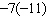a. 77 c. –77 b. 66 d. 88

2.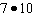a. 60 c. 80 b. –70 d. 70

3.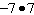a. –49 c. –77 b. –14 d. 49

4.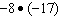a. 119 c. 136 b. –136 d. 153

5.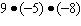a. 364 c. 362 b. 360 d. –360

6.

A manatee dives at a rate of 8 feet per second. What integer represents the change in the manatee’s position after 25 seconds?
 a. –200 c. –225 b. –195 d. 200

Evaluate the expression.

7.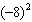a. 64 c. 16 b. –16 d. –64

8.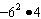a. 144 c. –144 b. 48 d. –48

9.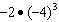a. –24 c. 24 b. 128 d. –128

Write an ordered pair corresponding to the point.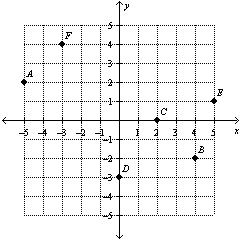10.

Point A
 a. (–2, 5) c. (–5, 2) b. (5, –2) d. (2, –5)

11.

Point E
 a. (5, 1) c. (–1, –5) b. (–5, –1) d. (1, 5)

Numeric Response

Multiply.

1.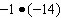Tell whether the product is positive or negative without multiplying. Explain your reasoning.

1.

8(–9)

2.

A quotient is undefined. What does this mean?